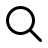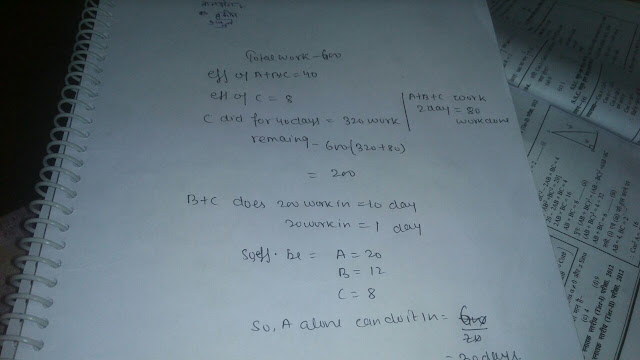# Knowledge Fusion- A Preparation Guide to Achieve|IBPS SBI RRB SSC exams

Daily Preparation Material & Quizzes for General Awareness,Computer,Banking,GS and many more...### Part1 Aptitude ssc rrb ibps Questions with solutions

All the stuff discussed in the Quant Whatsapp Group with question and solution are posted here in this post.Part 1 will be updated till it becomes 100 quant questions with solutions on this page.

 Mishra invested Rs.25,000 in two fixed deposits X and Y offering Compound interest at 6% per annum and 8% per annum, respectively. IF the total amount of interest accrued in two years through both fixed deposits is Rs. 3518 , then the amount invested in scheme X is ?
(Question posted by Divya. Time-13:50)

A)Rs.12000
B)Rs. 13500
C)Rs.15000
D)Cannot be Determined (CND)

Solution

Let the principle invested by A be "x" then the principle invested by B is 25,000-x
so the sum of interest should be 3518 after 2 years

x*2*6/100 + (25000-x)*2*6/100=3518
solving we get x = 12000

so the principle invested by A is 12,000 and that of B is 13,000



A,B and C can do a work in 15 days. After all the three worked for 2 days A left. B & C worked for 10 more days and B left. C worked for another 40 days and comeplete the work. In how many days A alone complete the work if C can complete it in 75 days? (posted by Divya)

Solution: By Atul

2/15+10(1/15-1/A)+40/75=1

On solving A we get A=30 days

OR
Solution Posted by Vinay shukla

OR
Solution posted by Mukesh

A + B = 1/15 - 1/75    ----(1)
2A + 12B = 1 - 52/75  -- (2)

Solving both
A= 30
B=50

OR

Solution Posted by Sarvesh

Thus B=50 days A=30 days


Three men A, B and C working together can do a job in 6 hours less time than A alone, in 1 hour less than time than B alone and in one half the time needed by C when working alone. Then A and B together can do the job in (Posted by Sarvesh)

Solution:

Posted by Atul..

1/x+6  + 1/x+1   + 1/2x  = 1/x

Solving X we will get

x=4/3

OR

Posted by ~

1/x + 1/x-6 + 1/2(x-6) = 1/x-6

Solve
Put the values of x in A nd B

Ans..4/3


A woman buys apples at 15 for a rupee and the same number at 20 a rupee. She mixes and sells them at 35 for 2 rupees. What is her gain per cent or loss per cent?(Posted by Atul)

Solution:

posted by (Atul)

(LCM of 15, 20 and 35) = 420 apples

Cost at 15 per rupee = 420/15 = 28

Cost at 20 per rupee = 420/20 = 21

Total cost for 840 apples = Rs. 49

SP for 840 apples =

840×2/35

= 48

Loss per cent =

1×100/49

= 2.04%.

OR

60@15=4
60@20=3

120 pe cost=7

120 ka sale price=120×2/35=240/35=6.85 aprx
So 0.15 loss which is aprx 2%


The ci on a certain sum for 2yrs is rs 756 and si is rs. 720 if the sum is invested such that the Si is rs. 900 and the number of yrs is equal to the rate per cent per annum find the r% - can anyone explain... posted by (~)

Solution:

Now, si =900
R=t
R*T= SI *100/P
= 900*100/3600
=25%
R=T= 5%

[6 ]Posted by Shivam

How many kilograms of sugar costing Rs. 9 per kg must be mixed with 27 kg of sugar costing Rs. 7 per kg so that there may be a gain of 10 % by selling the mixture at Rs. 9.24 per kg?

Solution:Posted by Mala

9      7
8.4
1.4     .6

Then  ratio 7:3
27/3=  9
7*9=  63

Ans: 63

=========================================

<<<<>>>>

Click here For part 2 ,  For part 3 Solved Aptitude Stuff

<<<<>>>>